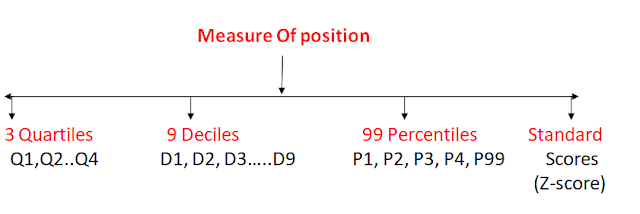# Measure of position

Measure Of position

Measure of position: A measure of position is a method by which the position that a particular data value has within a a given data set can be identified.

OR

Measure of position are used to locate the relative position of the data value in the data set.

The most common measure of position are  quartiles, deciles, percentiles and standard score()z score .

Suppose you have a data set 10,20,30,40,50,60

suppose mean =50.

consider any particular value say 10 check its relative position with mean.Q1-First quartile or (lower quartile)

Q2-Median

Q3-Third quartile or (upper quartile)

Quartiles: Divides the data in four equal parts.

Deciles: Divides the data in ten equal parts.

Percentiles: Divides the data in 100 equal parts.

3 Quartiles

Q1:-lower quartile is the value below which 25% obs’s lie.

Q1=(N+1)/4th item/obs’s.

Q2:Median is the value below which 50 obs’s lie

Q2=2(n+1)/4 th  item/obs’s.

Q3:Median is the value below which 50 obs’s lie

Q3= 3(n+1)/4 th  item/obs’s

For grouped Data

QK  =  K(N+1)/4 th term.

Q1 = l+(n/4-cf)/f*i

Q2 = l+(2N/4-cf)/f*i

Q3 = l+(3N/4-cf)/f*i

Q= l+(KN/4-cf)/f*I

9 Deciles:

D1 ,D2 , D3 …… D9 which divides the data into 10 equal parts .for discrete freq. dist. When arranged in ascending order.

Dk   = kth deciles

Dk=k(N+1/10)th item/obs.

For grouped data

Dk=l+(kN/10-c.f/f)*i)

K=1,2,3….9

99 Percentiles:

99 partition (position) values Will divide the data into 100 equal parts. Percentiles are denoted by p1,p2,p3….p99.

Kth percentiles, Pk=k(N+1/100)TH item/obs.

When data is arranged in ascending order.

For grouped  Data

Pk=l+(k.N/100-c.f)/f)*i

Ques.1-Find Q1,Q3,D7,P67

15,10,25,18,17,21,27,29,30

Sol.-Count the number.=9

Arrange the data in ascending order

10,15,17, 18, 21,25,27,29,30

N=9

Q1=(N+1)/4 th item

=(9+1)/4

=10/4

=2.5

2nd item+.5(3rd item-2nd item)

15+.5(17-15)

=15+.5(2)

16

Q3 =(3N+1)/4 th item

=3(9+1)/4

=7.5

=7TH item+ .5(8th -7th item)

=27+.5(29-27)

=28

D7= 7(N+1)/10th  term

D7=7(9+1/10)

=7

P67=67(n+1)/100th term

P67=67(9+1)/100

=67*10/100

=6.7

Ques.2-Find Q1,Q3,D7,P67

15,10,25,18,17,21,27,29,30

Sol.-Count the number.=9

Arrange the data in ascending order

10,15,17, 18, 21,25,27,29,30

N=9

Q1=(N+1)/4 th item

=(9+1)/4

=10/4

=2.5

2nd item+.5(4th item-3rd item)

15+.5(18-17)

=15+.5

15.5

Q3 =(3N+1)/4 th item

=3(9+1)/4

=7.5

=7TH item+ .5(8th -7th item)

=27+.5(29-27)

=28

D7= 7(N+1)/10th  term

D7=7(9+1/10)

=7

P67=67(n+1)/100th term

P67=67(9+1)/100

=67*10/100

=6.7

=25+.7(27-25)

=25+.7(2)

=26.4

Ques.3 Calculate the lower and upper quartile ,fourth deciles and 60 the percentiles from the following distribution.

 Marks group No. of student 05-10 5 10-15 6 15-20 15 20-25 10 25-30 5 30-35 4 35-40 2 40-45 2

Sol:- The cumulative frequency table is

 C.I f c.f 05-10 5 5 10-15 6 11 15-20 15 26 20-25 10 36 25-30 5 41 30-35 4 45 35-40 2 47 40-45 2 49

Here n=49, N/4= 12.25th term lies in 15-20 class

l=15, cf=11, f=15,  i=20-15=5

Q1=l+(N/4-cf)/f*i

=15+(12.25-11)/15*5

=15.41

ii)Q3=3/4N=36.75th term lies in  25-30 class

l=25,cf=36, f=5, i=5.

Q3= l+(3N/4-cf)/f*i

=25+(36.75-36)/5*5

=25.75

iii)D4=4N/10=4/10*49

=19.6th term lies in the class 15-20.

l=15,cf=11 , f=15,i=5

D4= l5+(4/10N-cf)/f*i

=15+((19.6-11)/15)*5

=17.92

iv)P60=60/100N=60/100*49=29.4th term lies in 20-25

l=20, cf=26, f=10, i=5

P60=l+((60/100N-cf)/f)*i

=20+(29.4-26)/10*5

=20+1.7

=21.7

Ques.4 The scores on a  midterm examination are presented below in decreasing order of   magnitude Find the percentile rank of a score of  63.

65,65,65,64,64,63,62,62,62,61,61,61,60,60,60,60,59,59,58,58,57,57,57,57,57,56,56,

56,48,47

Sol. We know that a percentile rank of k means, k % student has got marks below pk.

Here pk= 63

No of student whose marks are below 63.

Percentile rank=24/30*100=80%

It means 80% student got marks below Pk.

Standard score or (z score)

Standard score or (z score)-: A z-score is also known as standard score. Z-score are expressed in term of standard deviation from the mean.

-If a z-score is equal to zero, it is on the mean.

-if a z-score is equal to +1, it is one S.D above the mean.

-if  a z-score is equal to+2, it is 2 S.D above the mean.

Example: Suppose you got 75 marks ,average of the class is 50.

you have

+-σ =68..27%

+-2σ=95.45%

+-3σ =97.73%

In order to use  a z-score ,you need to know the mean ,μ and also the population s.d.

Formula of z -score

z=value-mean/s.d

Example: Raj score 85 in chemistry, while the class average was 80,with s.d of 5.vineet score 70 in his class, while average i was 60 with s.d 6. Who score better to their class.

Sol- For Raj

Z=x-μ /σ

z=85-80/5

z=5/5=1σ

For Vineet

z=70-60/6

=10/6

z=1.67σ

means vineet performed better than raj.

Example:-GMAT average score is 600 with S.D of 250.Find the standard score of  person who scores 750.

Sol.-Z=x-μ /σ

750-600/250

=150/200

=.60

The score is .605 above the mean

It means person not performed good.

Z score may also be =+ve and-ve,

With a +ve value indicating the score is above the mean and -ve score indicate it is below the score

Example:

Lets x=400, μ =500, σ 50

then Z=x-μ /σ

400-500/50=

-100/50

-2σ

Score is 2σ   below mean

The performance is 95% bad from the mean .we always measure this in term of σ not in term of no.

A z-score gives you an idea of how far from the mean a data pt. is. But more technically it is a measure how many s.d,'s below or above the population mean a raw score is.

Ex: Knowing that someone's wt. is 85 kg might

be good information, but if you want to compare it to the "avg" person's weight,

A z-score can tell you where that person's weight is compared to the avg population's mean weight.

Example:- Lets say

you have a test score of 190

the test has  μ =150

σ=25

Z=x-μ /σ

=1.6σ